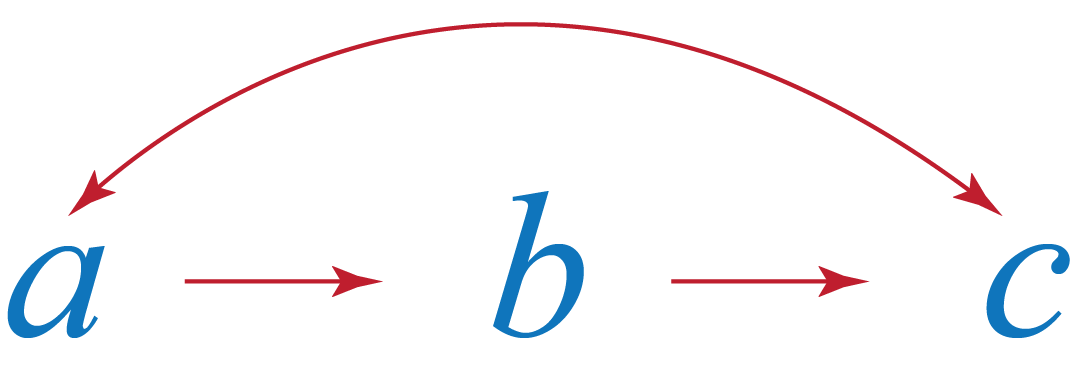Transitive Property

Transitive Property

Peter, James, and David went for a walk in the park. James kept a track of the distance he covered. David and James were walking together and hence covered the same distance. Peter said that he and David had also walked the same distance. Can we thus conclude that the distance Peter covered is equal to the distance James recorded?

You will get a better understanding of the above relationship in this section.

The chapter will explore the transitive property meaning, transitive property of equality, transitive property of angles, and transitive property of inequality.

Check out the interactive simulations on the concept of transitive property and try your hand at solving a few interesting practice questions at the end of the page.

Lesson Plan

 1 What is Transitive Property? 2 Important Notes on Transitive Property 3 Solved Examples on Transitive Property 4 Challenging Questions on Transitive Property 5 Interactive Questions on Transitive Property

What is Transitive Property?

Transitive means to transfer.

"If $$a$$, $$b$$, and $$c$$ are three quantities, and if $$a$$ is related to $$b$$ by some rule and $$b$$ is related to $$c$$ by the same rule, then $$a$$ and $$c$$ are related to each other by the same rule."

This property is called Transitive Property.If $$a$$, $$b$$, and $$c$$ are three numbers such that $$a$$ is equal to $$b$$ and $$b$$ is equal to $$c$$, then $$a$$ and $$c$$ are equal to each other.

Let's assume Mary ate 2 hotdogs and Jake ate as many as Mary.

This indicates that they ate the same number of hotdogs.

Let's have a better understanding of the transitive property meaning and see its application.

What is the General Formula of Transitive Property?

The formula for the transitive property of equality is

 If $$a = b \,$$ and $$\,b = c\,$$, then $$\,a = c$$

Here $$a$$, $$b$$, and $$c$$ are three quantities of the same kind.

For example, if $$a$$ is the measure of an angle, then $$b$$ or $$c$$ can't be the length of the segment.

Example: If $$x=m\,$$ and $$\,m=7 \,$$, then we can say $$\,x=7$$

The value 7 is transferred to $$x$$ because $$x$$ and $$m$$ are equal.

How to Use Transitive Property?

To use transitive property, we need three or more quantities for relating.

This property can be extended to the transitive property of angles and transitive property of inequality as well.

Transitive Property of Angles

If we have angles $$m$$ and $$n$$ such that $$m=n\,$$ and $$n=p\,$$ and $$\,m= 40^{\circ}$$, then by the transitive property of angles, we get $$p=40^{\circ}$$

Transitive Property of Inequality for Real Numbers

If we have three real numbers $$x$$, $$y$$, and $$z$$ such that, $$x \leqslant y \,$$ and$$\, y\leqslant z\,$$, then$$\, x\leqslant z$$.Similarly, if we have a number $$p \leqslant 5 \,$$ and$$\, 5\leqslant q\,$$, then$$\, p\leqslant q$$.

Use the transitive property of equality and inequality in the simulation below.

More Important Topics
Numbers
Algebra
Geometry
Measurement
Money
Data
Trigonometry
Calculus
More Important Topics
Numbers
Algebra
Geometry
Measurement
Money
Data
Trigonometry
Calculus
Learn from the best math teachers and top your exams

• Live one on one classroom and doubt clearing
• Practice worksheets in and after class for conceptual clarity
• Personalized curriculum to keep up with school